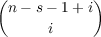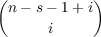#### Vol. 16, No. 3, 1966

 Download this articleFor screen For printingRecent Issues Vol. 325: 1  2 Vol. 324: 1  2 Vol. 323: 1  2 Vol. 322: 1  2 Vol. 321: 1  2 Vol. 320: 1  2 Vol. 319: 1  2 Vol. 318: 1  2Online Archive Volume: Issue:The Journal Subscriptions Editorial Board Officers Contacts Submission Guidelines Submission Form Policies for Authors ISSN: 1945-5844 (e-only) ISSN: 0030-8730 (print) Special Issues Author Index To Appear Other MSP Journals
New infinite classes of periodic Jacobi-Perron algorithms

### Leon Bernstein

Vol. 16 (1966), No. 3, 439–469
##### Abstract

The question whether a system of n 1 real algebraic numbers (n = 2,3,) chosen from an algebraic field of degree not higher than n, yields periodicity by Jacobi’s Algorithm is still as open and challenging as hundred years ago. The present paper gives an affirmative answer to this problem in the following case: let K(w) be an algebraic number field generated by w = (Dn d : m)1∕n, where m, n, d, D are natural numbers satisfying the conditions m 1, n 3, dD, 1 d D∕2(n 1). Then n 1 numbers can be chosen from K(w), so that their Jacobi Algorithm becomes purely periodic. The length of the period equals n2 (or n, if d = m = 1). This is the longest period of a periodic Jacobi Algorithm ever known. In three corollaries the following special cases are investigated

 w = (Dn − dr)1∕n, (r = 0,1,⋯,n) w = (Dn − drD)1∕n, (r = 0,1,⋯,n − 2) w = (Dn − pd∕m)1∕n. (n = pu,p a prime, u = 1,2,⋯,m as before)
In all these three cases the Algorithm of Jacobi remains purely periodic with length equal to n2.

The main tools in proving these results are the polynomials

 fs(w,D − 1) = ∑ 0sws−i(D − 1)i, Fs(w,D) = ∑ 0sws−iDi, (s = 1,⋯,n − 1)
of which each is an inverse function of the other.

Primary: 10.32
Secondary: 10.66
##### Milestones